## Question of the Week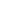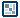Scripting 3D in Flash
by senocular

Trigonometry
So we've pretty much exhausted basic camera movement. It's time now to step it up a notch to camera rotation. Instead of changing position in space, this will be centered around changing the direction in which you're looking - effectively, camera rotation. For that, however, you'll need to know some trigonometry (screams mysteriously sound in the distance). It can get a little confusing if you don't already know it or at least the basics, so put your brain-caps on and prepare yourself because here we go. If you got trigonometry down pretty well already, you can probably skip this next part. Otherwise, I'll cover the basics of trig for those of you who may be in the dark. After all, it's a driving force for just about everything else in 3D that follows.

Trigonometry (or trig) is the branch of mathematics that deals with the relationships between the sides and the angles of triangles and the calculations based on them. What do triangles have to do with rotation? Well, when ever you have, for example, some line segment rotated at an angle in 2D space, it makes a triangle with the x and y axes on which it sits (or really the axes at which it exists - this doesn't always have to be the x and y as you'll soon see when it comes to 3D).

We'll assume the following. On the x, y axis there exists a line. This line is rotated where one end point of that line is at (0,0) or on the intersection of x and y axes and the other is off at some distance away in a diagonal location which would represent a rotation from the x axis. A triangle can then be made using that line with a horizontal vertical and line extending through and from the x axis.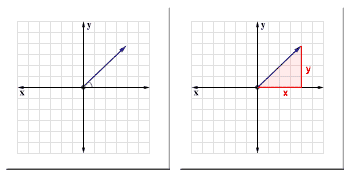[ a line drawn at an angle produces a triangle ]

Rotating this line from its current angle then changes lengths of those new lines that make up the sides of the triangle. These sides' lengths will fluctuate between a 0 and a length of the original line, which is also the hypotenuse of the triangle, all depending on the angle of rotation. One thing trig will be able to do for you, and what we'll need, is give you a way to find out the lengths of those lines through the use of the trig operations sine and cosine (Math.sin() and Math.cos() in Flash). These lengths will be important to us for positioing and, well rotation, of shapes and things within the scene.

Sine and cosine take in the angle of rotation of the original line as measured in radians and return a decimal percentage value of either the horizontal side along x (cosine) or the vertical side along y (sine) in relation to the original hypotenuse line's length. Multiplying the returned decimal percentage of each on the hypotenuse length will give you, respectively, the length of x and y, or really, coordinate of the end point (x,y) of that hypotenuse line since this line is being drawn form the point (0,0) or the origin.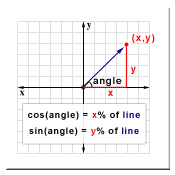[ sine and cosine to get x and y ]

With the calculation of this coordinate you know the end point of that line at any rotation. If you rotate a line around at a steady speed constantly and then set a movieclip in Flash to the position of the calculated (x,y) coordinate, you can easily have that clip follow in a circular path that's created by that rotation. The only variables Actionscript-wise are the angle, and the length of the rotating line. This length of the line (remember its also the hypotenuse of the triangle formed) is commonly referred to as "radius" as, in terms of the circle created in this rotation, that length represents the radius.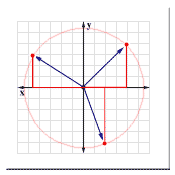[ circular path of line rotation ]

So in Flash, all you need to do is use the following equation.

Then the movieclip named "clip" will rotate around the x, y axis at a distance of radius based on the change in angle. Of course, as you might have guessed, this is all based around the point (0,0). And where is (0,0) in Flash? In the upper left. So for that, the origin offset is needed to put that rotation in a more centered portion of the screen giving you:

clip._x = originx + Math.cos(angle)*radius;
clip._y = originy + Math.sin(angle)*radius;

Setting that clip in motion is just a matter of, then, creating a constantly changing angle value - and remember, that angle is measured in radians, not degrees.

[ sine and cosine for rotation ]

And there you go, your basic commonly used set of equations for handling circular rotation in Flash using trig.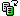There are a few different ways to measure angles. The most common, the one you are most familiar with, is the measurement of degrees. In terms of degrees, there are 360 degrees in a full circle. The Math functions sin and cos (sine and cosine) in Flash, however, use radians as an angle measurement. Though radians measure the same angles degrees do, they have smaller values to represent the same angle. Where there is 360 degrees in a full circle, there are 2 Pi radians. In Flash, 2 Pi would be 2*Math.PI. For conversion from one angle scale to another, you would simply use a multiplier fraction. This fraction is either just 2 Pi / 360 for degrees or 360 / 2 Pi to get radians. Using reduction, in getting rid of the 2, these can be reduced to Pi/180 and 180/Pi or Math.PI/180 and 180/Math.PI respectively. So to convert a degree angle into radians, use radians = degrees*Math.PI/180. For radians to degrees, you'd use degrees = radians*180/Math.PI. This may come in handy for future reference.

To go along with that sine and cosine malarkey, trig also provides a method to get the angle from your calculated x and y values. This is basically what was done before with sine and cosine but backwards. In Flash, the operation doing this takes on the form of Math.atan2(y,x). The atan stands for trig's arc tangent, and the 2 is just because there already is a prior arc tangent function Math.atan in Flash. Math.atan is still arc tangent, but instead of two x and y values, it takes a slope value which is the normal input for arc tangenet. Slope is simply a value derived from y divided by x. It gives you an indication of how steep a line is based on x and y values that for its triangle. Now, it is true you could just as well say Math.atan(y/x) in Flash instead of Math.atan2(y,x). However, built into atan2 is a check to return a proper value if x is 0. As we all know (or should know) you CANNOT divide by 0 and using an x of 0 in y/x ... well it's just not good. Math.atan2 lends a helping hand to prevent difficulties you'd otherwise encounter with that.

angle = Math.atan2(clip._y, clip._x); // angle based from (0,0)

One more thing you should be aware of is the Pythagorean theorem. The Pythagorean theorem will, when given your x and y values of your formed triangle, be able to give you the length of the hypotenuse; something which may also come in use now and then. Actually, you can obtain any length of any side as long as you have the length of the other 2. In Flash, that equation is handled as follows:

hypotenuse = Math.sqrt(x*x + y*y);
x = Math.sqrt(hypotenuse*hypotenuse - y*y);
y = Math.sqrt(hypotenuse*hypotenuse - y*y);

Because the radius in the sine and cosine equations is the hypotenuse of the triangle formed, if you don't know its value, the Pythagorean theorem here can be used to get that for you.

With a foundation of rotation concepts and equations in 2D, there can then be the jump into 3D. This jump actually isn't much of a jump ad all. More like a skip. After all, when it comes down to it, 3D is nothing more than 2D with an extra D. The same ideas for rotation apply. The only difference is that with that extra D they can be applied to a new set of coordinate spaces. With 2D, you only had x and y to work in. Now you have combinations of x, y; x, z and y, z. Rotation can be applied to any and all of these which would then give you... (drum roll please)... 3D rotation.